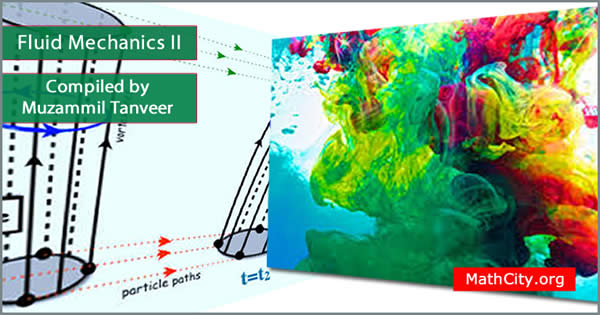# Fluid Mechanics II by Dr Rao Muzamal HussainThese notes are provided and composed by Mr. Muzammil Tanveer. We are really very thankful to him for providing these notes and appreciates his effort to publish these notes on MathCity.org. These notes are based on lectures delivered by Mr. Muzammil Hussain at GC University Faisalabad.

 Name Fluid Mechanics II Dr Rao Muzamal Hussain Muzammil Tanveer 76 pages PDF 783 kB
• Fluid
• Stress
• Sheer stress
• Normal stress
• Surface force
• Body force
• Newtonian and Non-Newtonian fluid
• Flow
• Density
• Viscosity
• Kinematic viscosity
• Compressibility
• Compressible fluid
• Incompressible fluid
• Ideal fluid
• Viscous fluid
• Rotational flow
• Irrotational flow
• Stream lines
• Potential line
• Laminar and Turbulent flow
• Stream lines
• Differential Equations of stream lines
• Vortex motion
• Vorticity vector
• Vortex line
• Vortex tube or Vortex filament
• Circulation
• Different types of Vortices
• Vortex pair
• Vortex Rows
• Flow along a curve
• Circulation
• Kelvins Theorem (For rotation or circulation) or State and prove Kelvins theorem for circulation
• Uniqueness Theorem
• Single Infinite Row of Vortices
• Double Infinite Row of Vortices
• Velocity Potential
• Kinetic Energy of Irrotational Motion
• Kelvin’s Minimum Energy Theorem
• Laplace Equation
• Normal stress
• Tangential stress or sheer stress
• Generalization equation of motion
• Euler equation of motion for in-viscus (real) fluid
• Bernoulli Equation
• Bernoulli Equation for steady flow
• Navier-Stokes equation
• Parallel flows
• Couette flow
• Generalization of Couette flow
• Plane Poiseuille flow
• Poiseuille flow or General Poiseuille flow
• Steady laminar flow through a circular pipe (The Hagen-Poiseuille flow)
• Couette-Poiseuille flow
• Flow between two concentric rotating cylinders
• Pressure distribution
• Maximum Velocity
• Shearing Stress
• Torque on the cylinder
• Flow through a cylinder of uniform cross-section
• Reynold Transport Theorem
• Transport of mass
• Transport of any dynamical

Please click on View Online to see inside the PDF.

• msc/notes/fluid-mechanics-ii-muzammil-tanveer